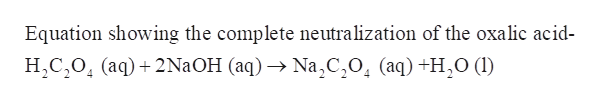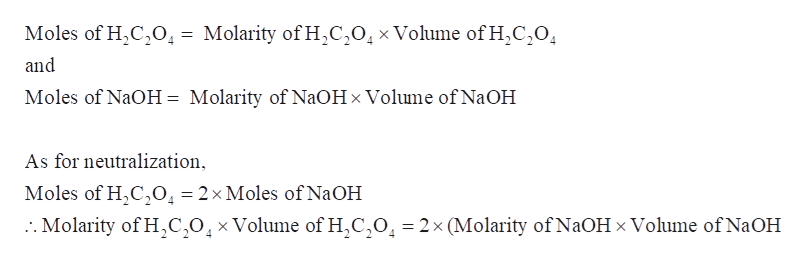# What volume (in mL) of the following acid would be required for complete neutralization of 50.00mL of 0.100 M NaOH?b. 0.100M Oxalic acid H2C2O4(aq), (a diprotic acid)

Question
1 views

What volume (in mL) of the following acid would be required for complete neutralization of 50.00mL of 0.100 M NaOH?

b. 0.100M Oxalic acid H2C2O4(aq), (a diprotic acid)

check_circle

Step 1

The volume (in mL) of the following acid that would be required for complete neutralization of 50.00mL of 0.100 M NaOH is to be calculated-

b. 0.100M Oxalic acid H2C2O4(aq), (a diprotic acid)

Step 2

Oxalic acid (H2C2O4) is a diprotic acid, thus it will require to 2 equivalents of NaOH to neutralize 1 equivalent of H2C2O4.help_outlineImage TranscriptioncloseEquation showing the complete neutralization of the oxalic acid- HC204 (aq)2N2OH (aq) Na,C,0, (aq) +H20 (1I) fullscreen
Step 3

Therefore, for complete neutralization:

Moles of ...help_outlineImage TranscriptioncloseMoles of H2C2O. = Molarity of H,C,01xVolume ofH2C20 and Moles of NaOH = Molarity of N2OH x Volume of NaOH As for neutralization, Moles of H,C,0, = 2x Moles of N2OH :. Molarity of HC,0,xVolume of H,C,0 2x (Molarity of NaOH x Volume of NaOH fullscreen

### Want to see the full answer?

See Solution

#### Want to see this answer and more?

Solutions are written by subject experts who are available 24/7. Questions are typically answered within 1 hour.*

See Solution
*Response times may vary by subject and question.
Tagged in

### General Chemistry